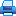Legal and postal addresses of the publisher: office 1410a, 17 Naberezhnaya Severnoy Dviny, Arkhangelsk, 163002, Russian Federation, Northern (Arctic) Federal University named after M.V. Lomonosov

Phone: (818-2) 21-61-21
E-mail: vestnik_est@narfu.ru
http://aer.narfu.ru/en/

# On the Eigenvalues of the Sturm– Liouville Operator with a Singular Potential. P. 115–125Section: Physics. Mathematics. Informatics

517.927

### Authors

T.A. Safonova*, S.V. Ryabchenko*
*Northern (Arctic) Federal University named after M.V. Lomonosov (Arkhangelsk, Russian Federation)
Corresponding author: Tat’yana Safonova, address: Naberezhnaya Severnoy Dviny, 17, Arkhangelsk, 163002, Russian Federation; e-mail: t.Safonova@narfu.ru

### Abstract

The asymptotic behavior of the eigenvalues and eigenfunctions depending on the coefficients of the differential expression as well as the regularized trace formulas for the corresponding operators are highly relevant in the modern spectral theory of differential operators. In the case of the Sturm–Liouville operator with a continuously differentiable potential the basic results were obtained by I.M. Gelfand and B.M. Levitan in 1953. Later, in the works of L.A. Dikiy, V.A. Sadovnichiy, V.B. Lidskiy, V.A. Marchenko and other mathematicians, these results were extended for the case of the higher orders differential operators and partial differential operators. A.A. Shkalikov and A.M. Savchuk first considered similar issues in 1999–2003 for the Sturm–Liouville operator with a singular potential, which was not locally integrable function, and Dirichlet boundary conditions on a finite interval. In the relatively recent works of A.G. Kostyuchenko and S.R. Ismagilov (2007–2008) the leading term of the asymptotics of the counting function for the self-adjoint extensions of the Sturm-Liouville vector operator generated by the expression l[ y] = ‑y′′(x) +Q(x) y(x) in the space L22 (R+) was obtained, where Q(x) – a real symmetric square matrix of the second order.This paper is devoted to finding the transcendental equations for the eigenvalues of the self-adjoint operator generated by the expression 1 1 [ ]( ) ( ) ( ) ( ) n k k k l y x y x h x x y x ‑ = = ‑ ′′ +Σ d ‑ , where k x k n = , k h ∈R (k =1,2,...,n ‑1), and d(x) – d - delta function, and separated boundary conditions of the form y(0) = y(1) = 0, y(0) = y (1) = 0, y (0) = y (1) = 0 in the space L2[0,1] . The further analysis of received equations allows us to obtain the asymptotics of the eigenvalues and the first order regularized trace formula for these operators.

### Keywords

quasiderivative, Sturm–Liouville operator with a singular potential, eigenvalue